# NCERT Solutions for Class 9 Maths Chapter 6 - Lines and Angles

Share

The NCERT Solutions For Class 9 Maths Chapter 6 – Lines and Angles have been made available here in the simplest of manner. These NCERT Solutions have been prepared by the expert teachers in compliance with the updated syllabus of the Class 9 CBSE. Thus, by solving our Class 9 Maths Chapter 6 Solutions, students can get familiar with the important topics of the Chapter and gain more confidence going into the exam.

Chapter 6 of the Class 9th Maths deals with the topic Lines and Angles. Further, it goes on to explain critical topics such as Basic Terminology, Intersecting Lines And Non-Intersecting Lines, Pairs of Angles as well as Lines Parallel To The Same Line among others. All these topics are explained here in a detailed manner using examples and illustrations which assist students to develop clarity over certain topics. So, by utilizing our NCERT Solutions for Class 9 Chapter 6, you can very easily prepare the Chapter and perform well in the exam.

## NCERT Solutions for Class 9 Maths Chapter 6 - Lines and Angles

Exercise 6.1
• Exercise 6.1
• Exercise 6.2
• Exercise 6.3
Class 9th|NCERT - MathematicsChapter 6 - Lines and Angles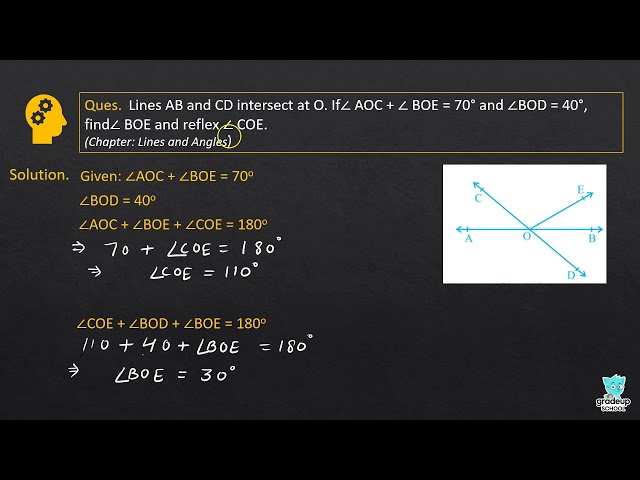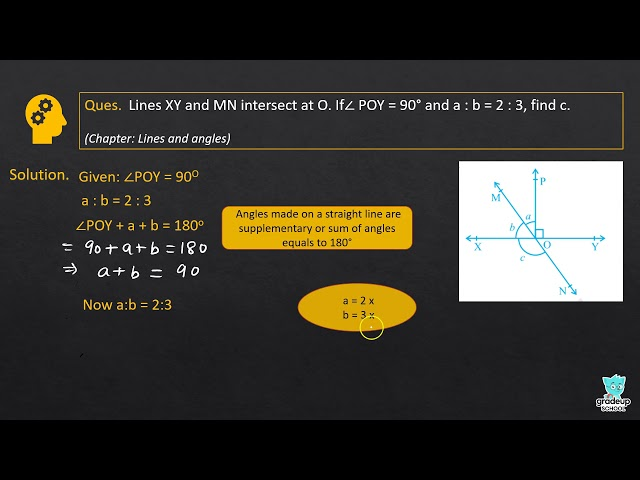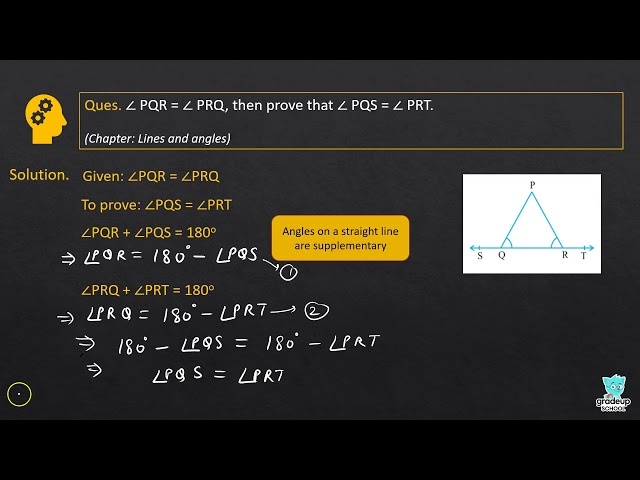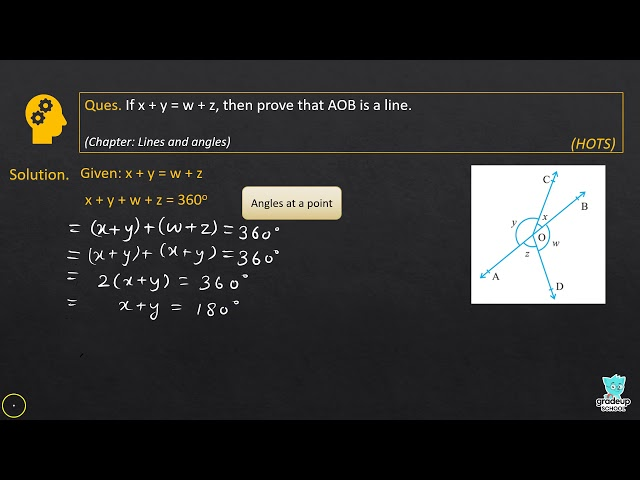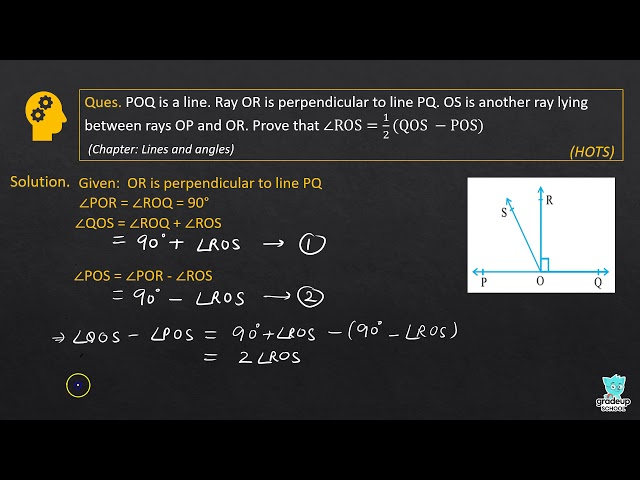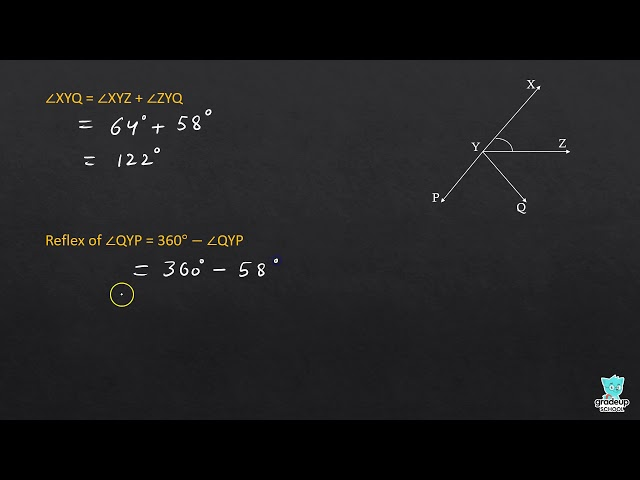NCERT - MathematicsClass 9th , NCERT
 Chapter 1 - Number System Chapter 2 - Polynomials Chapter 3 - Coordinate Geometry Chapter 4 - Linear Equations in two Variables Chapter 5 - Introduction to Euclid's Geometry Chapter 6 - Lines and Angles Chapter 7 - Triangles Chapter 8 - Quadrilaterals Chapter 9 - Areas of Parallelograms and Triangles Chapter 10 - Circles Chapter 11 - Constructions Chapter 12 - Heron's Formula Chapter 13 - Surface Areas and Volumes Chapter 14 - Statistics Chapter 15 - Probability